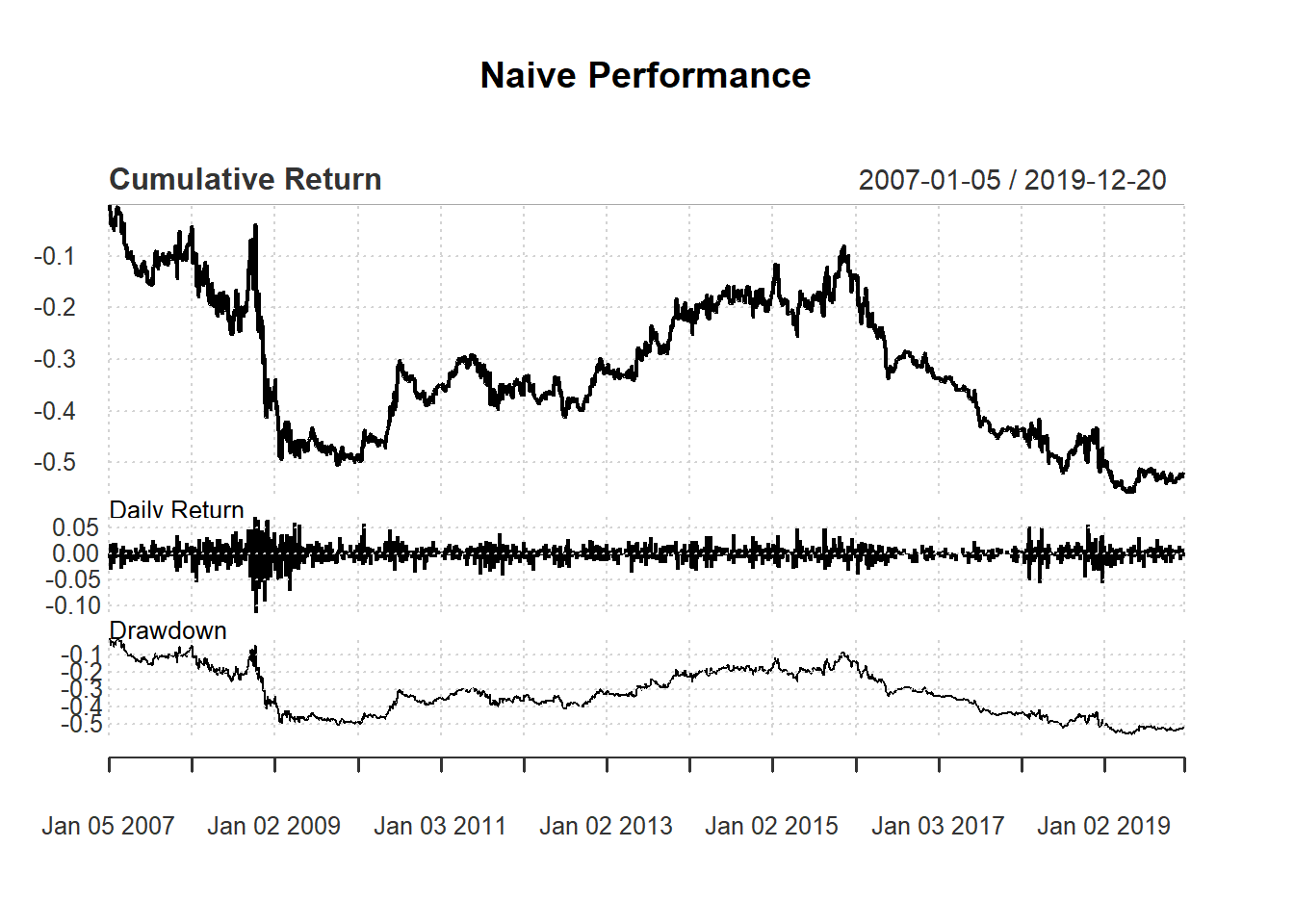Here we create trading signal based on simple filter rule. Recall that simple filter rule suggests buying when the price increases a lot compared to the yesterday price and selling when price decreases a lot: \begin{align*} \text{Buy}&:\frac{P_t}{P_{t-1}}>1+\delta \\ \text{Sell}&:\frac{P_t}{P_{t-1}}<1-\delta \end{align*}

where $$P_t$$ is the closing price at time $$t$$ and $$\delta>0$$ is an arbitrary threshold.

library(quantmod)
getSymbols("MSFT")
##  "MSFT"

price <- Cl(MSFT)
r <- price/Lag(price) - 1
returnMSFT <- (Cl(MSFT) - Op(MSFT))/Op(MSFT)

delta<-0.005
signal <-c(NA) # first signal is NA

for (i in 2: length(Cl(MSFT))){
if (r[i] > delta){
signal[i]<- 1
} else if (r[i]< -delta){
signal[i]<- -1
} else
signal[i]<- 0
}
signal<-reclass(signal,Cl(MSFT))

To keep thing simple, we allow costless short selling. Otherwise, we only allow a sell after a buy.

trade <- Lag(signal)
charts.PerformanceSummary(ret)